# The Association Rules In Data Science

0
376Association rules finding is a rule-based machine learning technique used widely in recommender systems. If you have seen advertisements that are tailored according to your interests and preferences, chances are association rules were used to show you that specific advertisement. Association rule mining is principally the process of finding correlations between data points in a data set. The ‘rules’ here are the conditions used to specify the occurrence of a particular data point in a set given the occurrence of other data points. It finds which data points are the most correlated and are hence more likely to occur together. Essentially it learns rules of the form ‘If X occurs, Y will occur’. Each rule has some thresholds and parameters which specify how likely the rule is going to be true and other such properties. We then select the rules which are the best representative of the data and which are most suited for the task at hand.

To give a clearer picture, let’s say we have a dataset of the purchases made by the customers in a supermarket. We see that 70% percent of the customers who bought peanut butter also bought jelly (which is a popular combination used in sandwiches). Based on this dataset, we can then form an association between peanut butter and jelly such as ‘If a customer buys peanut butter, he will also buy jelly’. But this rule is not always true because we saw that only 70% of the customers followed this rule and the other 30% didn’t. Hence, we specify some properties of association rules that help us determine the efficacy of the rules. These are also called measures of interestingness since they help us determine which rules are interesting and are thereby useful.

This kind of association rules helps us determine several interesting correlations between sets in the dataset. Some of the associations are not-so-obvious ones and help significantly in market-oriented approaches, say for supermarkets to predict which items to bring in; or to show users advertisements specific to their tastes and preferences. One interesting and famous correlation found from association rule forming is the correlation between beer and diapers being bought together frequently in supermarkets. This seems counter-intuitive correlation was later explained by the fact that new fathers are tasked with shopping, while the mothers are left with the baby. As this example goes to show that this sort of rule forming can reveal associations that are not obvious and can significantly help markets in providing a better experience to the users.

## Formalization Of Association Rules

We define the formal definition of association rules and their properties as follows:

Given a database of transactions D = {t1,t2,t3,….,tn} in which each transaction has a subset of the set of the set of all items I = {i1,i2,i3….,im} we try to find rules of the form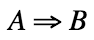such that A,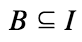This means that we are finding rules that relate subsets of the item set based on our observations as stored in the dataset. In the ruleA is called the antecedent and B is called the consequent. In our previous example, peanut butter is the antecedent and jelly is the consequent.

There are several measures of interestingness which help us determine the usefulness of a rule. We list some of them below:

Support

The support of an antecedent, A determines the frequency of the set A in the database D. That is to say, it the fraction of transactions t in which A is present with respect to the database D.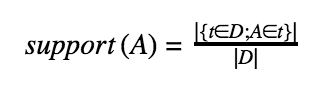Note that the support of A decreases as the size of A increases, since an increase in size leads to an increase in restrictions.

Confidence

The confidence of a ruleis the proportion of the transactions in D which contain both the sets A and B. It determines the likelihood of a rule being true.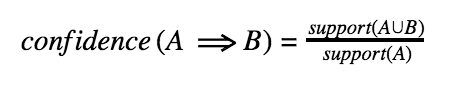Lift

Lift determines the efficiency of the rule as a whole taking into consideration both the confidence of the rule and the database. It is defined as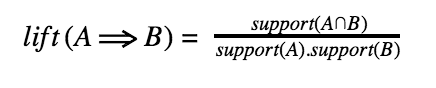It is a measure of the dependencies of A and B on each other. If both of them are independent of one another, the lift will have a value of 1. This is because the lift is essentially the ratio of the probability of A and B occurring together and the probability of them occurring together if they were independent. This gives a comprehensive evaluation of the correlation and dependencies between A and B.

Finding Association Rules

Finding the association rules for a given dataset leads to interesting correlations in the dataset. Once we have the association rules, we can apply it to predict the occurrence of any set given the occurrence of the sets it is associated with. In practice, association rules are found by first finding the frequent items in a dataset; that is, the items which have a possibility of correlation. This is done by using a minimum support threshold, that is, by discarding the sets which have a support less than a specific, predefined value. Then, we can form rules to all the associations by using confidence as a measure to filter out the associations with confidence less than a predefined value.

There are many algorithms to find out the association rules from a dataset. We’ll discuss the most commonly used algorithm called the Apriori Algorithm. This finds out the frequent items list given a minimum support threshold and a database T. It is then straightforward to apply the confidence threshold to generate association rules.

It uses a breadth-first search technique to determine the number of possible frequent items. It uses a candidate list of length k to determine a candidate list of length k+1 . It then searches for the frequent items from the candidate list. The pseudo-code for the algorithm is given below.